Maths Course in English

# Simple Interest Formulas: Short Tricks and Questions

Simple Interest Formulas: Short Tricks and Questions: In this page, you will learn about percentage Problems, Short Tricks in English. This topic is very important for every 2019 competitive exams preparation. So before proceeding for any exam read these short tricks and explanations carefully about Simple Interest Questions with Short Tricks with the explanation.

## Simple Interest with Example

Simple Interest is a fee paid by a borrower of assets to the owner as a form of compensation for the use of the assets. It is most commonly the price paid for the use of borrowed money or money earned by deposited funds.

When money is borrowed, interest is typically paid to the lender as a percentage of the principal, the amount owed to the lender. The percentage of the principal that is paid as a fee over a certain period of time (typically one month or year) is called the interest rate. A bank deposit will earn interest because the bank is paying for the use of the deposited funds. Assets that are sometimes lent with interest include money, shares, consumer goods through hire purchase, major assets such as aircraft, and even entire factories in finance lease arrangements. The interest is calculated upon the value of the assets in the same manner as upon money.

If the interest is paid as it falls due, at the end of the decided period (yearly, half yearly or quarterly) the principal is said to be lent or borrowed at simple interest.

### Quicker Method to solve the Questions

Simple Interest (SI)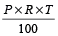Here P = principal, R = rate per annum, T = time in years

Therefore Amount (A)If T is given in months, since rate is per annum, the time has to be converted into years, so the period in months has to be divided by 12. If T = 2 months this is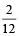years)

Example 1: Find the simple interest and amount when Rs. 1000 is lent at 5% per annum for 5 years.

Solution: By the formula,

SI =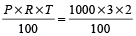= Rs. 60

∴ Amount = P + SI = 100 + 60 = Rs. 1060

Principal =Example 2: Find the principal when simple interest is Rs. 60 at 4% per anum for 4 years.

Solution:

Principal =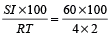= Rs. 750

Time =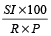Example 3: In how many years will the sum of Rs. 500 become Rs. 620 if the rate of simple interest is 4% per annum?

Solution: Using the formula,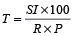Here, SI = 620 – 500 = Rs. 120

∴= 6 years

Rate =Example 4: At what rate percent per annum will a sum of money double in 8 years?

Solution: Let principal = Rs. P,Then SI = Rs. P and Time = 8 years

∴ Rate =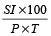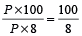% per annum